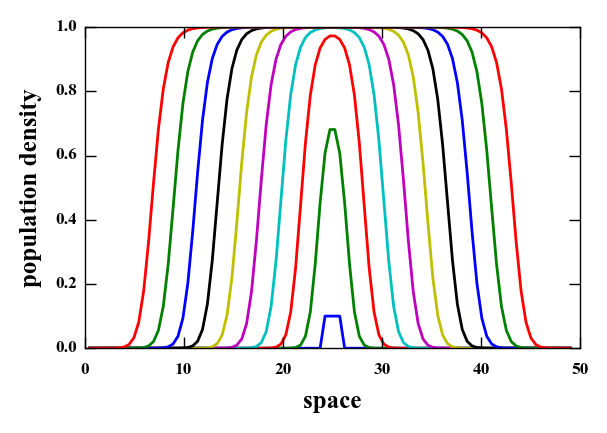## A simple implementation of the Fisher-Kolmogorov¶

We will show below a simple method to numerically solve the Fisher-Kolmogorov equation, by using a technique known as the "method of lines", which involves discretizing the space domain in equally-spaced intervals and turning the partial differential equation (PDE) into a (large) system of ordinary differential equations (ODEs).

it is important to remember that methods to solve PDEs are considerably more involved than for ODE, and there is no single method for all equations. Also, depending on the type of PDE, the description of the problem (such as initial conditions, boundary conditions etc.) can be quite different and impose further restrictions.

In :
from numpy import *
from scipy.integrate import odeint
% matplotlib inline
from matplotlib.pyplot import plot, xlabel, ylabel

# parameters
r = 1.
K = 1.
D = 0.1

# the size of the spatial domain
# his is actual size, such as "kilometres"
L = 50.
# the number of points in the grid
grid_size = 100
# the integration times
t = arange(0, 300, 0.1)
# the grid
dx = L / (grid_size+1)
grid = arange(0, L, dx)[1:-1]

# the initial condition, consisting of a small "square" in the middle
y0 = zeros_like(grid)
y0[grid_size//2 - 2:grid_size//2 + 2] = 0.1

# let's define the flux
def fkpp(y, t, r, K, D, dx):
# we calculate the spatial second derivative
d2x = -2 * y
d2x[1:-1] += y[2:] + y[:-2]
d2x += y
d2x[-1] += y[-2]
d2x = d2x/dx/dx
# then add the reaction terms
dy = r * y * (1. - y/K) + D * d2x
return dy

y = odeint(fkpp, y0, t, (r, K, D, dx))

In :
# let us plot the solution

for i in linspace(t, t[-1], 10):
plot(grid, y[int(i),:])
xlabel('space')
ylabel('population density')

Out:
<matplotlib.text.Text at 0x7f727175aac8>In [ ]:
# now for some real fun! let's animate the solution!

# THIS WILL NOT WORK IN THE NOTEBOOK
# you have to copy this into an ipython shell and run it there

def animate(grid, data, skip_frames=1, labelx='x', labely='', labels=[], log=False):
import matplotlib
# this is required. In case of problems, try one of:
# GTK, GTKAgg, TkAgg, WX, WXAgg
matplotlib.use('GtkAgg')
from pylab import plot, legend, xlabel, ylabel, ion, draw, ylim, yscale
import time

ion()

nvars = shape(data)//len(grid)
ldata = [ data[:,i*len(grid):(i+1)*len(grid)] for i in range(nvars) ]
lines = []
for d in ldata:
lines.append(plot(grid, d))
xlabel(labelx)
ylabel(labely)
if len(labels) == nvars:
legend(labels)
ymin = 0 if data.min() > 0 else floor(data.min())
ylim((ymin, ceil(data.max())))
if log:
yscale('log')
ylim((1e-25, 15))
for l in range(len(lines)):
lines[l].set_ydata(ldata[l])  # initial condition
draw()
time.sleep(0.1)
tstart = time.time()               # for profiling
for i in range(1, shape(data)//skip_frames):
for l in range(len(lines)):
lines[l].set_ydata(ldata[l][i*skip_frames])  # update the data
draw()                         # redraw the canvas

print('FPS:' , shape(data)/(time.time()-tstart))

animate(grid, y)

In [ ]: